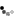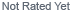Loading... Please wait...

Currency Displayed in

# What Is The Square Footage Of My Inground Swimming Pool?

## What Is The Square Footage Of My Inground Swimming Pool?

What is the Square Footage of my Inground Swimming Pool?

This formula is approximate and for Rectangle or Square Inground pools but should work for determining the amount paint needed to finish a pool. We recommend rounding up on the measurements and we always recommend getting an extra gallon of paint just in case.
You don't want to run out of paint in the middle of your project.

The formula is as follows:

(L x W) + (L x Avg Depth x 2) + (W x shallow depth) + (W x deep depth) =
Total square footage of all the pool sides and bottom.

To determine average depth in a pool where the bottom slopes: Measure the shallow end and measure the deep end. Add them together and divide by 2. This is the average depth of your pool.

Below is an example using a 16 x 32 pool with a Shallow depth of 4', a deep depth of 8' and an average depth of 6' feet

L x W = (32 x 16) = 512
L x Avg. Depth x 2 = (32 x 6 x 2) = 384
W x Shallow Depth = (16 x 4) = 64
W x Deep Depth = (16 x 8) = 128

512 sqft for the bottom + 384 sqft (the two long sides) + 64 sqft (small side) + 128 sqft (deep side) = 1,088 SQFT. Round up to 1,100 SQFT

To calculate the SQFT of a Round Pool, the formula is:

Example: 24' Round Pool - The Radius is 12',   12 x 12 x 3.14 = 452.16 or 452 SQFT

If you have any questions please feel free to give us a call at 1-800-876-7647

## Featured Products

•••••Return Policy

Shipping Policy